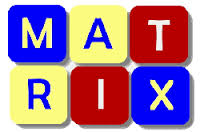# An easy IIT-JEE problem

Probability Level 3Let A be the set of all 3 × 3 symmetric matrices all of whose entries are either 0 or 1. Five of these entries are 1 and four of them are 0.

The number of matrices in A is?

×